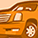# Power Management ICs Forum DiscussionsLevel 1Level 1

# BTN8982TA Current calculation

I am using BTN8982TA IC. In that IC, i am getting voltage as 1.93 in IS pin, and i am using Rs  as 3.6K.

How to calculate IL load current by using these values?

How to find the dkilis value?

1 SolutionModeratorModerator

# Re: BTN8982TA Current calculation

Hi,

We can calculate il and dkilis as follows,

ils = Vis/Ris  = 1.93/3.6x10^3 = 0.5361 x 10^-3 = 0.5361mA.

dkilis = 1/ils = 1865.3236

Load current = il = dkilis * ( ils - isoffset)

= 0.6828 A

Regards,

Abhilash P

3 RepliesModeratorModerator

# Re: BTN8982TA Current calculation

Hi,

We can calculate il and dkilis as follows,

ils = Vis/Ris  = 1.93/3.6x10^3 = 0.5361 x 10^-3 = 0.5361mA.

dkilis = 1/ils = 1865.3236

Load current = il = dkilis * ( ils - isoffset)

= 0.6828 A

Regards,

Abhilash PLevel 1Level 1

# Re: BTN8982TA Current calculation

HI Abilash,

what's the value of is-offset?.. So that we can calculate the load current.

Thanksyou.ModeratorModerator

# Re: BTN8982TA Current calculation

Hi,

Ioffset is 170uA.